# AP Physics B : Understanding Momentum and Impulse

## Example Questions

### Example Question #1 : Newtonian Mechanics And Motion

Two astronauts, Ann and Bob, conduct a collision experiment in a weightless, frictionless environment. Initially Ann moves to the right with a momentum of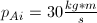, and Bob is initially at rest. In the collision, the two astronauts push on each other so that Ann's final momentum is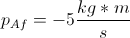to the left. What is Bob's final momentum?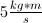to the rightto the left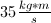to the right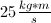to the leftto the rightto the right

Explanation:

Apply conservation of momentum before and after the collision.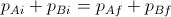.

Taking left to be the negative direction, and noting that Bob's initial momentum is 0 since he is at rest, we can use the provided information to see that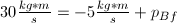.

Solving for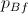, we get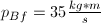. Since this answer is positive, Bob's momentum is in the positive direction (to the right).